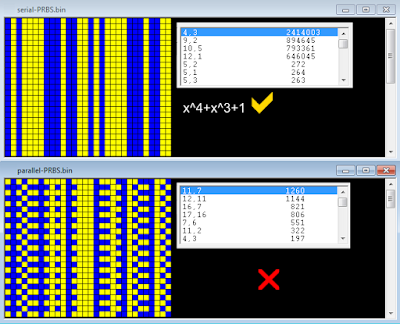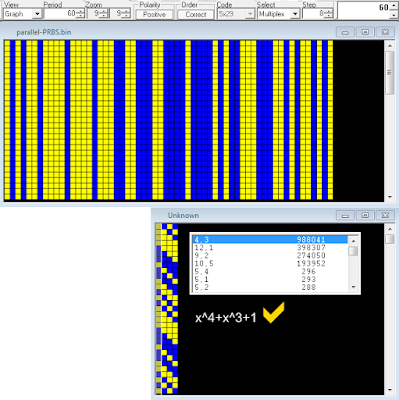### LFSR/PRBS

Last update:  28th June, 2022

Linear feedback shift registers (LFSRs), or their software implementations, are used to generate pseudo-random binary sequences (PRBS, also referred to as pseudo-random bit sequences) which are largely used in telecommunication (data scrambling, sync patterns, preambles, field delimiter markers, jamming, stream ciphers, bit-error rate tests, ...).
The feedback is formed by XORing or XNORing the outputs of selected stages of the shift register - referred to as taps - and then inputting this to the least significant bit (stage 0). The list of the taps is known as the tap sequence. It is this feedback that causes the register to loop through repetitive sequences of pseudo-random  value.
LFSRs are described by their characteristic polynomial: for example a tap sequence of [4, 3, 0] (the 0 corresponds to the x0 = 1 and can be omitted) describes the  polynomial x^4+x^3+1 (a 4-bit shift-register):

Below a table of the polynomials  you may find in this blog is shown along with the systems where they are employed; the LFSR polynomials used in STANAG, MIL-STD, and FED standards are not listed.

 polynomial referring post x^31+x^3+1 KW-46 encryption, sync x^18+x^13+x^11+x^5+x^2+1 Chinese Ny PSK2 2400 Bd serial x^13+x^9+x^7+1 AKKORD SS-PD-datalink, scrambler x^12+x^10+x^9+x^3+1 150-300Bd/900 FSK-2, sync x^11+x^9+1 CIS 81-81 T-206 x^9+x^8+x+1 MoD RK, 188-110A serial x^9+x^5+1 100-50Bd/500 FSK-2 CIS-12 (T-230) 318KBd/228 GFSK Rus PSK4 1200Bd CIS-1200 (T-230-1A) x^8+x^6+x^5+x^4+1 AKKORD SS-PD-datalink, sync x^8+x^6+x+1 SAAB GRINTEG MHF 50 CIS-75 (128-bit) x^7+x^6+1 CIS-75 126-bit French-Ny 50Bd/850 FSK-2 x^7+x^5+x+1 50Bd/500 FSK-2 x^7+x^4+1 Unid x^7+x^3+x^2+x+1 150-300Bd/900 FSK-2, CRC polynomial x^7+x^3+1 Swiss Army TC-535 x^6+x^5+1 French-Ny 50Bd/850 FSK-2 300Bd/500 FSK-2 STANAG-4481F x^5+x^4+x+1 50Bd/500 FSK-2 200Bd/400 MFSK-4 x^5+x^4+1 CIS-11 idle signal β x^4+x^3+1 CIS-Ny "Akula" x^3+x^2+x+1 256-bit Initialization Vectors encryption system x^3+x^2+1 CIS-11 idle signal α

Any PRBSn sequence will have a word length of n bits and a max sequence length of 2^n - 1 bits (also termed period T). The maximum periodicity of the sequence can only be achieved through XOR-ing only some combinations of a few particular stages of the LFSR: if the produced sequence  has the maximum periodicity  (T=2^n -1, maximum-length sequence) then that sequence is known as m-sequence and its characteristic polynomial is termed primitive polynomial:

There can be more than one maximum-length sequence for a given LFSR length. Also, once one m-sequence has been found, another automatically follows. If the tap sequence in an n-bit LFSR is [n, A, B, C] then the corresponding "mirror" sequence is [n, nA, nB, nC].  So the tap sequence [6, 5] has as its counterpart [6, 1], ie in terms of polynomials x^6+x^5+1 --> x^6+x+1 --> x^6+x^5+1.
With short sequence lengths the pattern is repeated many times, which has the effect of reducing the randomness of the signal, especially at higher bit rates. Long sequence lengths offer more randomness, however they have the disadvantage of taking a longer time to complete the entire sequence, which can be a issue at lower bit rates.
Notice that although a PRBS sequence demonstrates random behaviour, it is in fact generated deterministically and the bit sequence (short or long) will always be the same when repeated. By the way, a truly random sequence would be unusable, since receiving equipment would have no idea what was actually sent.

Another interesting approach
Sometimes it may happen to come across sync sequences or particular patterns of which it is not possible to trace back to the generator polynomial and therefore to the structure of the used LFSR. This happens because most of the time PRBS are drained from the serial output of the LFSR and therefore the search for the generator polynomial takes place brute-force and "directly" on the pattern being analyzed.Fig. 1 - x^4+x^3+1 LFSR with serial output

But it could happen that the pseudo-random sequence is instead formed by taking the outputs (the states) of all the stages that compose the LFSR, let's say a parallel-output PRBS as an alternative counterpart to the serial-output one.Fig. 2 - x^4+x^3+1 LFSR with parallel outputs

In the first case (serial-output PRBS) the sequence has a length (or period T) of 15 bit while in the second case (parallel-output PRBS) the sequence has a period  of 15×4=60 bit length. Analyzing the latter as if it were a serial-output one could produce not significant or even misleading results, as shown in figure 3.Fig. 3 - results after analyzing the serial and parallel output of the same LFSR (x^4+x^3+1)

What we need is establish the number of stages that compose the unknown LFSR, after which a single stage can be isolated and its (serial) output sequence analyzed. Well, assuming T is the period of the pattern, this one shall be reshaped to a c-columns array where c = T/((2^n)-1), where n = (2,3,4,5,6,7...).  After reshaped the original pattern, the search for the generator polynomial can be performed on any of the c columns of that array.  In a few words, we have to found the valor the period T in table below (if any) and then reshape the pattern according to the corrispondent value of c (ie, the number of the stages of the LFSR):

In case of the x^4+x^3+1 LFSR mentioned above, its parallel output has a period length of 60 bit and  is divisible by 15, giving 4 as the number of the stages: the polynomial is then easily verified after reshaping the 60-bit pattern to a 4-bit array (figure 4). Notice that the the length of the serial-output PRBS is just equal to 15.Fig. 4

Other examples are shown below:

Obviously, the above is valid only for certain lengths of the period T and it is not certain that it is also valid for generator polynomials that are not primitive, i.e. they do not generate sequences of maximum length . Moreover, it could be argued that a multiplexer is needed to send the parallel-output PRBS, but the whole sequence could also be stored and then pre-loaded and added to the bitstream as many times as needed, or it could happen that only a segment of a parallel-output PRBS could be used.

I am definitely not a "mathematician" but I think that sometimes the above method, though a bit raw, can be useful to unearth the LFSR which is hidden behind particular sequences.